# Expanded Genetic Graph

WWW Graph of Genetic for Link Analysis Ranking Experiments

## Examples

### Basic Examples

Retrieve the graph:

 In:=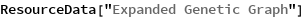Out=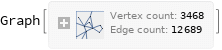Summary properties:

 In:=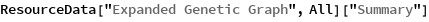Out=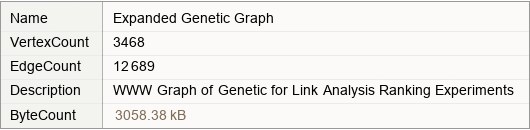### Basic Applications

Show the power-law degree distribution of the graph:

 In:=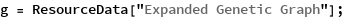In:=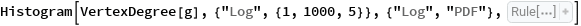Out=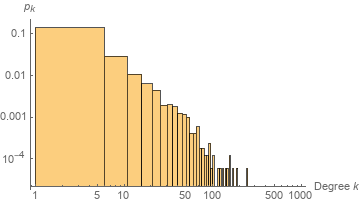Show a table of properties:

 In:=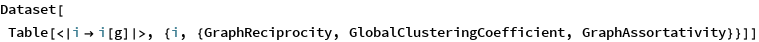Out=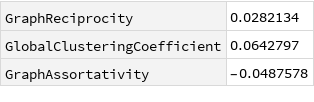Wolfram Research, "Expanded Genetic Graph" from the Wolfram Data Repository (2019)